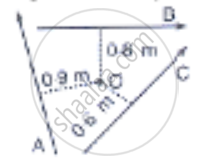Share

# A, B and C Are the Three Forces Each of Magnitude 4 N Acting in the Plane of the Paper as Shown in Figure. the Point O Lies in the Same Plane. - ICSE Class 10 - Physics

#### Question

A, B and C are the three forces each of magnitude 4 n acting in the plane of the paper as shown in Figure. The point O lies in the same plane.1) Which force has the least moment about O? Give a reason.

2) which force has the greatest moment about O? Give a reason.

3) Name the forces producing (a) Clockwise (b) anticlockwise moments.

4) what is the resultant torque about the point O?

#### Solution

1) We know that

Moment of Force  = Force x Perpendicular distance

Since the perpendicular distance of vector C is least from the point O.

Vector C will have the least moment about O.

2) We know that

Moment of force = Force x Perpendicular distance

Since the perpendicular distance of vector A is greatest from the point O.

Vector A will have the greatest moment about O.

3.a) Vector A and B will produce clockwise moments.

Explanation: if the turning effect on the body is clockwise.

The moment if force is called clockwise moment and it is taken as negative.

3.b) Vector C will produce anticlockwise moment.

Explanation L if the turning effect on the body is anticlockwise.

Moment of force is called anticlockwise moment and it is taken as positive.

4) Resultant torque about point O = sum of torques due to vectors A, B, and C

= -(4 xx 0.9) - (4 xx 0.8) + (4 xx 0.6) Nm